# Programming with Recursion Using Recursion 1 The Recursion

• Slides: 24Programming with Recursion Using Recursion 1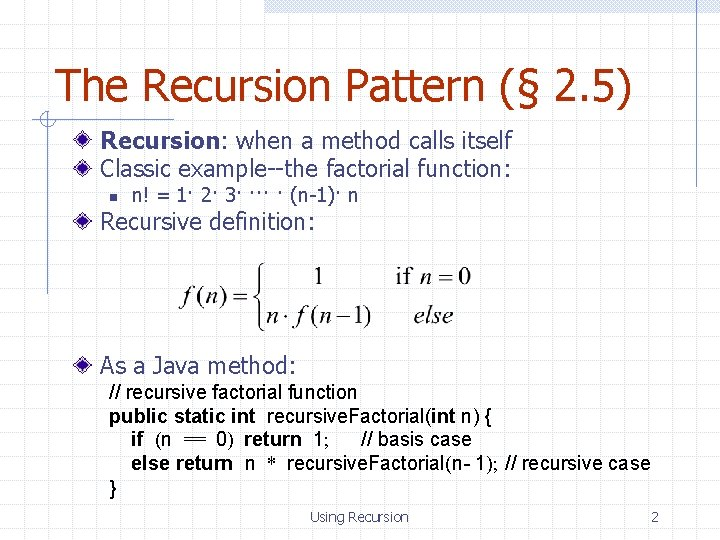The Recursion Pattern (§ 2. 5) Recursion: when a method calls itself Classic example--the factorial function: n n! = 1· 2· 3· ··· · (n-1)· n Recursive definition: As a Java method: // recursive factorial function public static int recursive. Factorial(int n) { if (n == 0) return 1; // basis case else return n * recursive. Factorial(n- 1); // recursive case } Using Recursion 2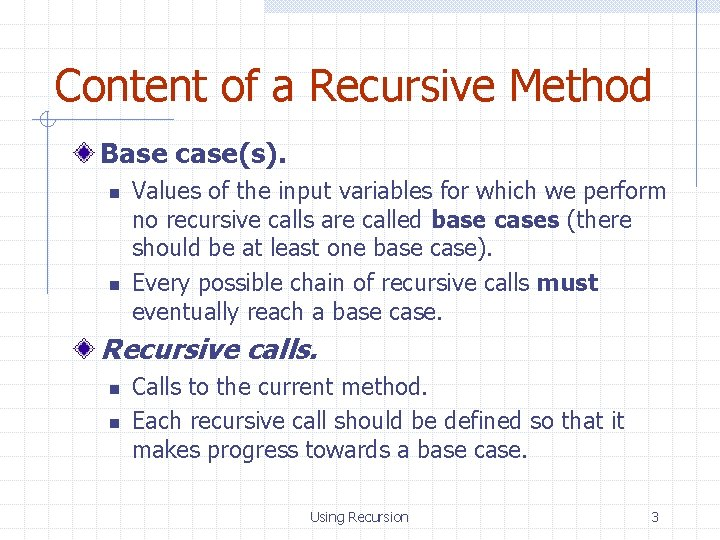Content of a Recursive Method Base case(s). n n Values of the input variables for which we perform no recursive calls are called base cases (there should be at least one base case). Every possible chain of recursive calls must eventually reach a base case. Recursive calls. n n Calls to the current method. Each recursive call should be defined so that it makes progress towards a base case. Using Recursion 3Visualizing Recursion Example recursion trace: Recursion trace A box for each recursive call An arrow from each caller to callee An arrow from each callee to caller showing return value return 4*6 = 24 call final answer recursive. Factorial(4) return 3*2 = 6 call recursive. Factorial(3) return 2*1 = 2 call recursive. Factorial(2) call return 1*1 = 1 recursive. Factorial(1) Using Recursion call return 1 recursive. Factorial(0) 4Example – English Rulers Define a recursive way to print the ticks and numbers like an English ruler: Using Recursion 5A Recursive Method for Drawing Ticks on an English Ruler // draw a tick with no label public static void draw. One. Tick(int tick. Length) { draw. One. Tick(tick. Length, - 1); } // draw one tick public static void draw. One. Tick(int tick. Length, int tick. Label) { for (int i = 0; i < tick. Length; i++) System. out. print("-"); if (tick. Label >= 0) System. out. print(" " + tick. Label); System. out. print("n"); } public static void draw. Ticks(int tick. Length) { // draw ticks of given length if (tick. Length > 0) { // stop when length drops to 0 draw. Ticks(tick. Length- 1); // recursively draw left ticks draw. One. Tick(tick. Length); // draw center tick draw. Ticks(tick. Length- 1); // recursively draw right ticks } } public static void draw. Ruler(int n. Inches, int major. Length) { // draw ruler draw. One. Tick(major. Length, 0); // draw tick 0 and its label for (int i = 1; i <= n. Inches; i++) { draw. Ticks(major. Length- 1); // draw ticks for this inch draw. One. Tick(major. Length, i); // draw tick i and its label } } Using Recursion 6Visualizing the Draw. Ticks Method An interval with a central tick length L >1 is composed of the following: n n n draw. Ticks (3) an interval with a central tick length L-1, a single tick of length L, an interval with a central tick length L-1. Output draw. Ticks (2) draw. Ticks (1) draw. Ticks (0) draw. One. Tick (3) draw. Ticks (2) (previous pattern repeats ) Using Recursion 7Using Recursion 8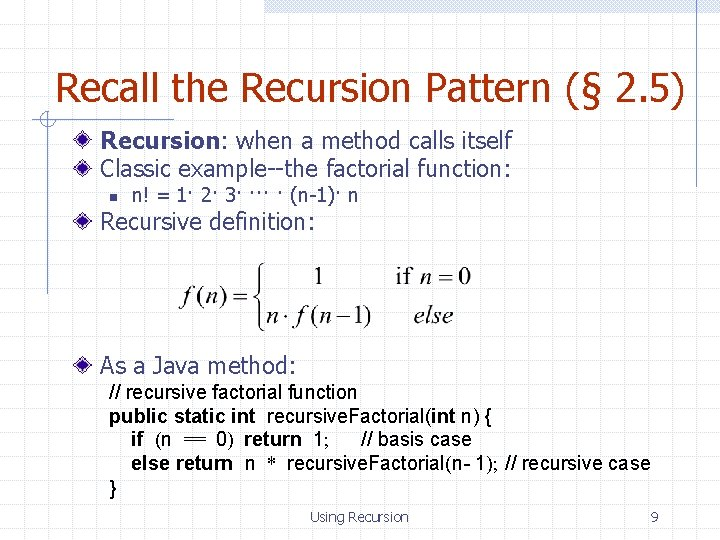Recall the Recursion Pattern (§ 2. 5) Recursion: when a method calls itself Classic example--the factorial function: n n! = 1· 2· 3· ··· · (n-1)· n Recursive definition: As a Java method: // recursive factorial function public static int recursive. Factorial(int n) { if (n == 0) return 1; // basis case else return n * recursive. Factorial(n- 1); // recursive case } Using Recursion 9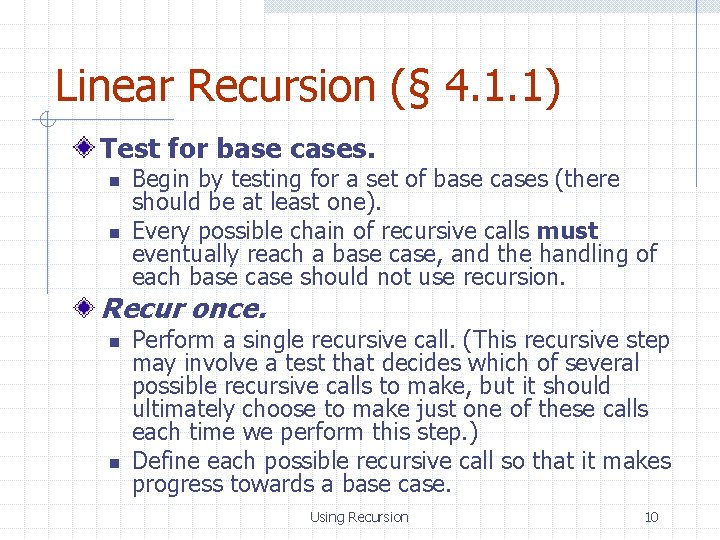Linear Recursion (§ 4. 1. 1) Test for base cases. n n Begin by testing for a set of base cases (there should be at least one). Every possible chain of recursive calls must eventually reach a base case, and the handling of each base case should not use recursion. Recur once. n n Perform a single recursive call. (This recursive step may involve a test that decides which of several possible recursive calls to make, but it should ultimately choose to make just one of these calls each time we perform this step. ) Define each possible recursive call so that it makes progress towards a base case. Using Recursion 10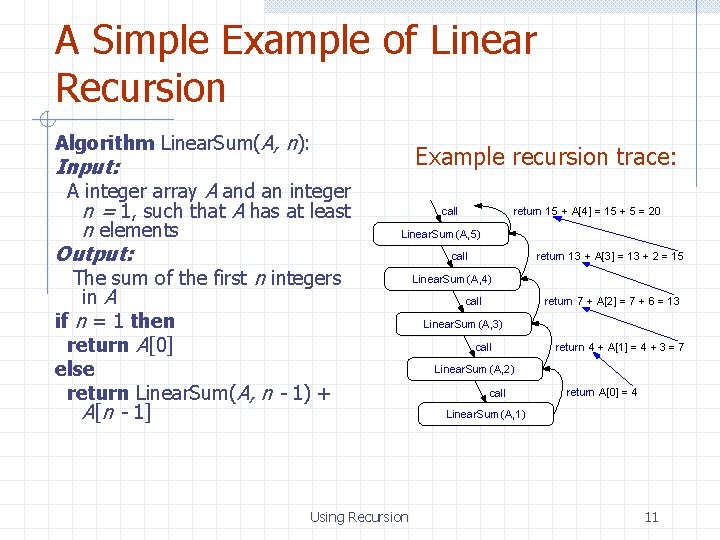A Simple Example of Linear Recursion Algorithm Linear. Sum(A, n): Example recursion trace: Input: A integer array A and an integer n = 1, such that A has at least n elements Output: call return 15 + A = 15 + 5 = 20 Linear. Sum (A, 5) call The sum of the first n integers in A if n = 1 then return A else return Linear. Sum(A, n - 1) + A[n - 1] Using Recursion return 13 + A = 13 + 2 = 15 Linear. Sum (A, 4) call return 7 + A = 7 + 6 = 13 Linear. Sum (A, 3) call return 4 + A = 4 + 3 = 7 Linear. Sum (A, 2) call return A = 4 Linear. Sum (A, 1) 11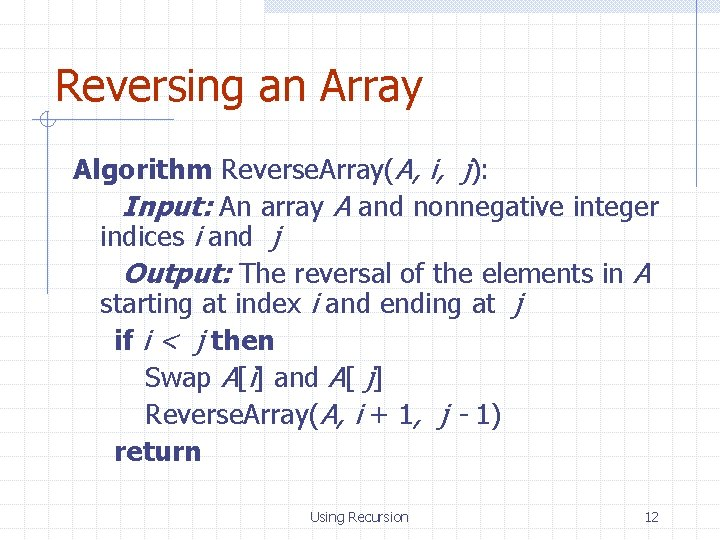Reversing an Array Algorithm Reverse. Array(A, i, j): Input: An array A and nonnegative integer indices i and j Output: The reversal of the elements in A starting at index i and ending at j if i < j then Swap A[i] and A[ j] Reverse. Array(A, i + 1, j - 1) return Using Recursion 12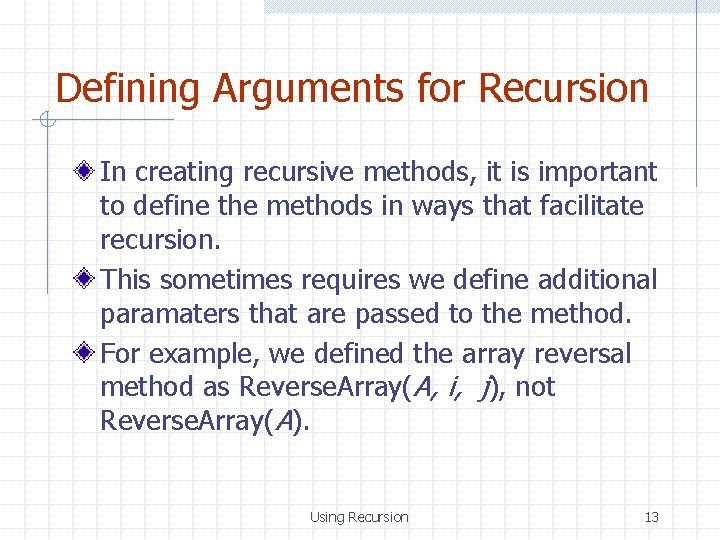Defining Arguments for Recursion In creating recursive methods, it is important to define the methods in ways that facilitate recursion. This sometimes requires we define additional paramaters that are passed to the method. For example, we defined the array reversal method as Reverse. Array(A, i, j), not Reverse. Array(A). Using Recursion 13Computing Powers The power function, p(x, n)=xn, can be defined recursively: This leads to an power function that runs in O(n) time (for we make n recursive calls). We can do better than this, however. Using Recursion 14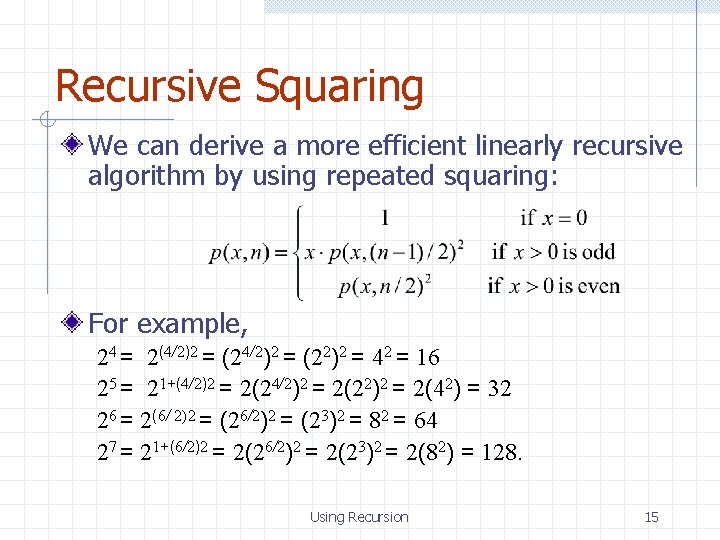Recursive Squaring We can derive a more efficient linearly recursive algorithm by using repeated squaring: For example, 24 = 2(4/2)2 = (22)2 = 42 = 16 25 = 21+(4/2)2 = 2(22)2 = 2(42) = 32 26 = 2(6/ 2)2 = (26/2)2 = (23)2 = 82 = 64 27 = 21+(6/2)2 = 2(23)2 = 2(82) = 128. Using Recursion 15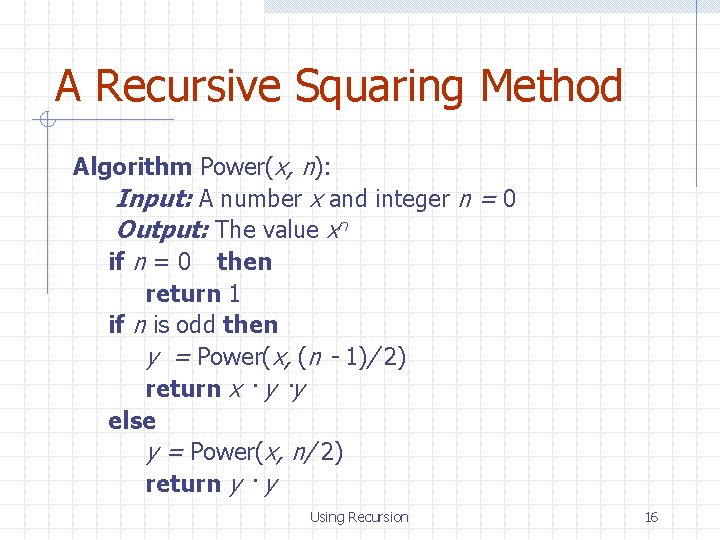A Recursive Squaring Method Algorithm Power(x, n): Input: A number x and integer n = 0 Output: The value xn if n = 0 then return 1 if n is odd then y = Power(x, (n - 1)/ 2) return x · y ·y else y = Power(x, n/ 2) return y · y Using Recursion 16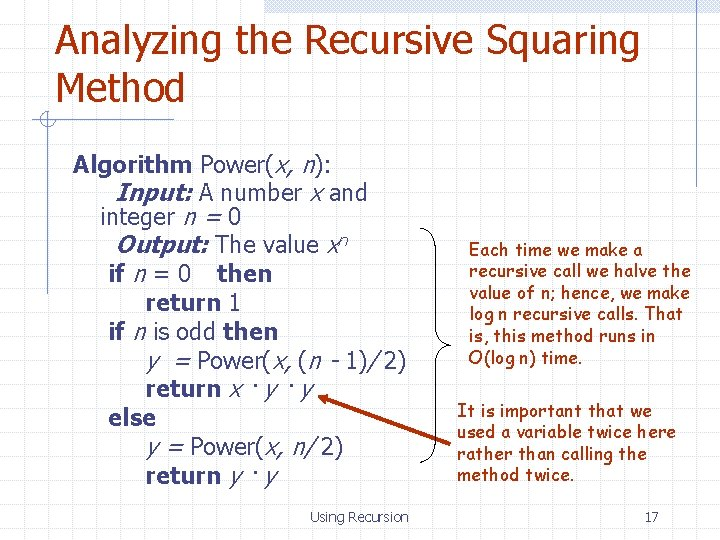Analyzing the Recursive Squaring Method Algorithm Power(x, n): Input: A number x and integer n = 0 Output: The value xn if n = 0 then return 1 if n is odd then y = Power(x, (n - 1)/ 2) return x · y else y = Power(x, n/ 2) return y · y Using Recursion Each time we make a recursive call we halve the value of n; hence, we make log n recursive calls. That is, this method runs in O(log n) time. It is important that we used a variable twice here rather than calling the method twice. 17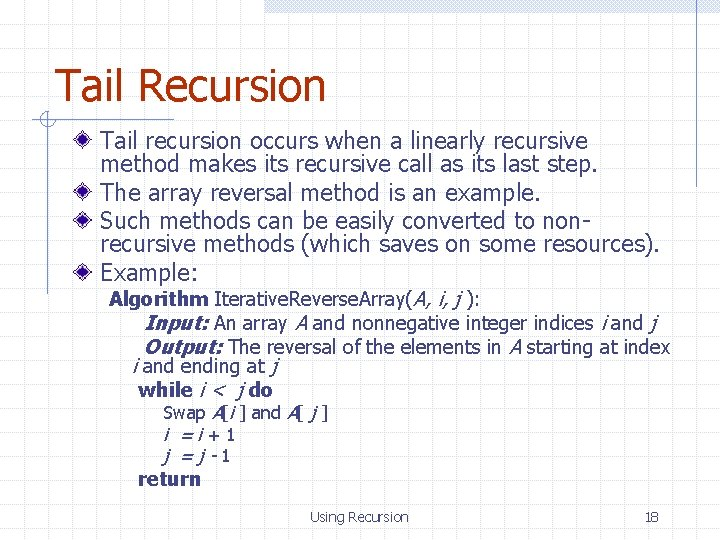Tail Recursion Tail recursion occurs when a linearly recursive method makes its recursive call as its last step. The array reversal method is an example. Such methods can be easily converted to nonrecursive methods (which saves on some resources). Example: Algorithm Iterative. Reverse. Array(A, i, j ): Input: An array A and nonnegative integer indices i and j Output: The reversal of the elements in A starting at index i and ending at j while i < j do Swap A[i ] and A[ j ] i =i+1 j =j-1 return Using Recursion 18Binary Recursion (§ 4. 1. 2) Binary recursion occurs whenever there are two recursive calls for each non-base case. Example: the Draw. Ticks method for drawing ticks on an English ruler. Using Recursion 19A Binary Recursive Method for Drawing Ticks // draw a tick with no label public static void draw. One. Tick(int tick. Length) { draw. One. Tick(tick. Length, - 1); } // draw one tick public static void draw. One. Tick(int tick. Length, int tick. Label) { for (int i = 0; i < tick. Length; i++) System. out. print("-"); if (tick. Label >= 0) System. out. print(" " + tick. Label); System. out. print("n"); } public static void draw. Ticks(int tick. Length) { // draw ticks of given length if (tick. Length > 0) { // stop when length drops to 0 draw. Ticks(tick. Length- 1); // recursively draw left ticks draw. One. Tick(tick. Length); // draw center tick draw. Ticks(tick. Length- 1); // recursively draw right ticks } } public static void draw. Ruler(int n. Inches, int major. Length) { // draw ruler draw. One. Tick(major. Length, 0); // draw tick 0 and its label for (int i = 1; i <= n. Inches; i++) { draw. Ticks(major. Length- 1); // draw ticks for this inch draw. One. Tick(major. Length, i); // draw tick i and its label } } Using Recursion Note the two recursive calls 20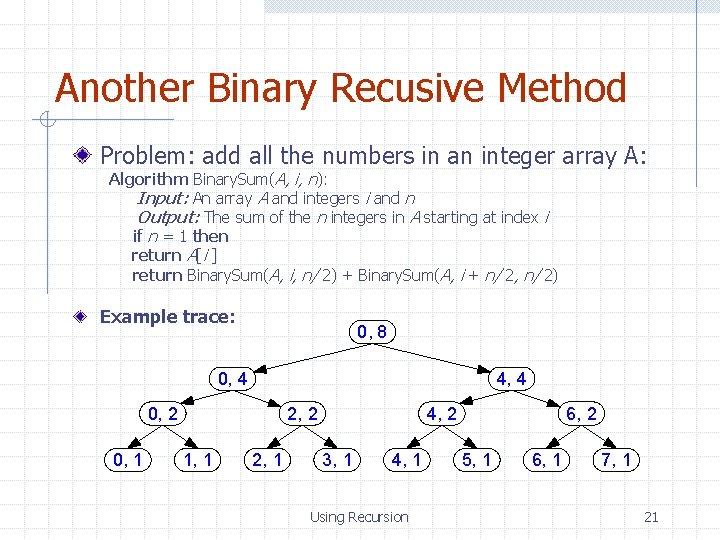Another Binary Recusive Method Problem: add all the numbers in an integer array A: Algorithm Binary. Sum(A, i, n): Input: An array A and integers i and n Output: The sum of the n integers in A starting at index i if n = 1 then return A[i ] return Binary. Sum(A, i, n/ 2) + Binary. Sum(A, i + n/ 2, n/ 2) Example trace: 0, 8 0, 4 4, 4 0, 2 0, 1 2, 2 1, 1 2, 1 4, 2 3, 1 4, 1 Using Recursion 6, 2 5, 1 6, 1 7, 1 21Computing Fibanacci Numbers Fibonacci numbers are defined recursively: F 0 = 0 F 1 = 1 Fi = Fi-1 + Fi-2 for i > 1. As a recursive algorithm (first attempt): Algorithm Binary. Fib(k): Input: Nonnegative integer k Output: The kth Fibonacci number Fk if k = 1 then return k else return Binary. Fib(k - 1) + Binary. Fib(k - 2) Using Recursion 22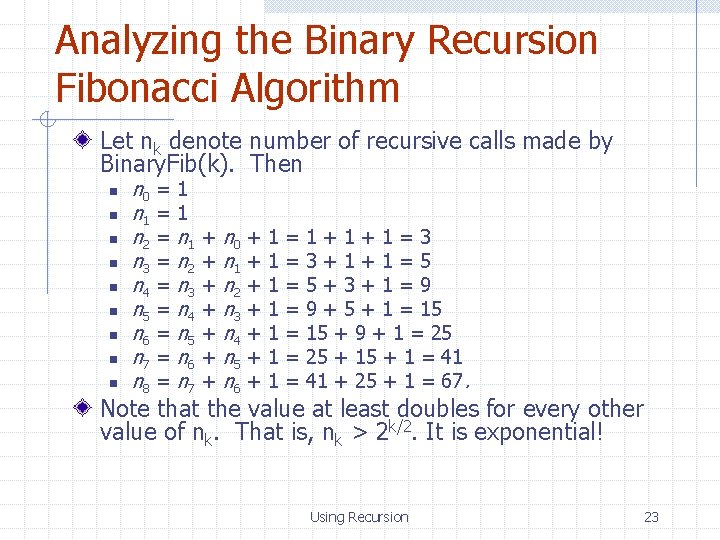Analyzing the Binary Recursion Fibonacci Algorithm Let nk denote number of recursive calls made by Binary. Fib(k). Then n n 0 = 1 n 1 = 1 n 2 = n 1 + n 0 + 1 = 1 + 1 = 3 n 3 = n 2 + n 1 + 1 = 3 + 1 = 5 n 4 = n 3 + n 2 + 1 = 5 + 3 + 1 = 9 n 5 = n 4 + n 3 + 1 = 9 + 5 + 1 = 15 n 6 = n 5 + n 4 + 1 = 15 + 9 + 1 = 25 n 7 = n 6 + n 5 + 1 = 25 + 1 = 41 n 8 = n 7 + n 6 + 1 = 41 + 25 + 1 = 67. Note that the value at least doubles for every other value of nk. That is, nk > 2 k/2. It is exponential! Using Recursion 23A Better Fibonacci Algorithm Use linear recursion instead: Algorithm Linear. Fibonacci(k): Input: A nonnegative integer k Output: Pair of Fibonacci numbers (Fk, Fk-1) if k = 1 then return (k, 0) else (i, j) = Linear. Fibonacci(k - 1) return (i +j, i) Runs in O(k) time. Using Recursion 24